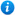* Watermarks do not appear on purchased resources

Preview resource: Click slides to enlarge

# Multiply By Ten

KS1 Maths Teaching Resources: Multiply by Ten

Year 2 maths programme of study - Number (multiplication and division):

• recall and use multiplication and division facts for the 2, 5 and 10 multiplication tables

In 'Multiply by Ten ' pupils practise the x 10 multiplication table.

'Multiply by Ten ' is an animated PowerPoint presentation which includes:

• interactive activities
• x 10 flash cards
• 2 worksheets

'Multiply by Ten ' can be fully edited allowing teachers to adapt, alter and revise it as much or as little as needed.

To preview 'Multiply by Ten' please click on the PowerPoint images.

Our Price : £1.99 / 2 Credits